xHankinson's equationEncyclopedia
Hankinson's equation is a mathematical relationship for predicting the off-axis uniaxial compressive strength of wood. The formula can also be used to compute the fiber stress
Stress (physics)
In continuum mechanics, stress is a measure of the internal forces acting within a deformable body. Quantitatively, it is a measure of the average force per unit area of a surface within the body on which internal forces act. These internal forces are a reaction to external forces applied on the body...

or the stress
Stress (physics)
In continuum mechanics, stress is a measure of the internal forces acting within a deformable body. Quantitatively, it is a measure of the average force per unit area of a surface within the body on which internal forces act. These internal forces are a reaction to external forces applied on the body...

wave velocity at the elastic limit as a function of grain
Wood grain
In speaking of wood the term grain refers to the alternating regions of relatively darker and lighter wood resulting from the differing growth parameters occurring in different seasons . The term is used in several ways. Perhaps most important is that in woodworking techniques...

angle in wood
Wood
Wood is a hard, fibrous tissue found in many trees. It has been used for hundreds of thousands of years for both fuel and as a construction material. It is an organic material, a natural composite of cellulose fibers embedded in a matrix of lignin which resists compression...

. For a wood that has uniaxial compressive strengths ofparallel to the grain and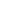perpendicular to the grain, Hankinson's equation predicts that the uniaxial compressive strength of the wood in a direction at an angleto the grain is given by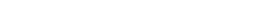Even though the original relation was based on studies of spruce
Spruce
A spruce is a tree of the genus Picea , a genus of about 35 species of coniferous evergreen trees in the Family Pinaceae, found in the northern temperate and boreal regions of the earth. Spruces are large trees, from tall when mature, and can be distinguished by their whorled branches and conical...

, Hankinson's equation has been found to be remarkably accurate for many other types of wood. A generalized form of the Hankinson formula has also been used for predicting the uniaxial tensile strength of wood at an angle to the grain. This formula has the form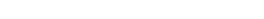where the exponentcan take values between 1.5 and 2.

The stress wave velocity at angle angleto the grain at the elastic limit can similarly be obtained from the Hankinson formula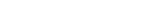where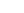is the velocity parallel to the grain,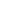is the velocity perpendicular to the grain andis the grain angle.

• Failure theory (material)
Failure theory (material)
Failure theory is the science of predicting the conditions under which solid materials fail under the action of external loads. The failure of a material is usually classified into brittle failure or ductile failure . Depending on the conditions most materials can fail in a brittle or ductile...

• Linear elasticity
Linear elasticity
Linear elasticity is the mathematical study of how solid objects deform and become internally stressed due to prescribed loading conditions. Linear elasticity models materials as continua. Linear elasticity is a simplification of the more general nonlinear theory of elasticity and is a branch of...

• Hooke's law
Hooke's law
In mechanics, and physics, Hooke's law of elasticity is an approximation that states that the extension of a spring is in direct proportion with the load applied to it. Many materials obey this law as long as the load does not exceed the material's elastic limit. Materials for which Hooke's law...

• Orthotropic material
Orthotropic material
An orthotropic material has two or three mutually orthogonal twofold axes of rotational symmetry so that its mechanical properties are, in general, different along each axis. Orthotropic materials are thus anisotropic; their properties depend on the direction in which they are measured...

• Transverse isotropy
Transverse isotropy
A transversely isotropic material is one with physical properties which are symmetric about an axis that is normal to a plane of isotropy. This transverse plane has infinite planes of symmetry and thus, within this plane, the material properties are same in all directions...